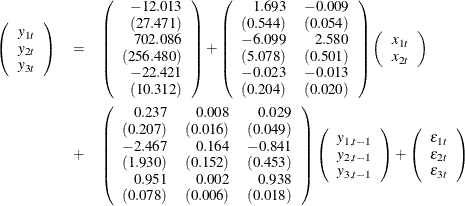# The VARMAX Procedure

### Vector Autoregressive Process with Exogenous Variables

A VAR process can be affected by other observable variables that are determined outside the system of interest. Such variables are called exogenous (independent) variables. Exogenous variables can be stochastic or nonstochastic. The process can also be affected by the lags of exogenous variables. A model used to describe this process is called a VARX(p,s) model.

The VARX(p,s) model is written as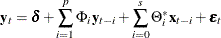where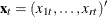is an r-dimensional time series vector and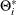is a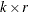matrix.

For example, a VARX(1,0) model is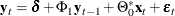where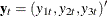and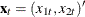.

The following statements fit the VARX(1,0) model to the given data:

data grunfeld;
input year y1 y2 y3 x1 x2 x3;
label y1='Gross Investment GE'
y2='Capital Stock Lagged GE'
y3='Value of Outstanding Shares GE Lagged'
x1='Gross Investment W'
x2='Capital Stock Lagged W'
x3='Value of Outstanding Shares Lagged W';
datalines;
1935  33.1 1170.6  97.8 12.93  191.5   1.8
1936  45.0 2015.8 104.4 25.90  516.0    .8
1937  77.2 2803.3 118.0 35.05  729.0   7.4
1938  44.6 2039.7 156.2 22.89  560.4  18.1

... more lines ...


/*--- Vector Autoregressive Process with Exogenous Variables ---*/

proc varmax data=grunfeld;
model y1-y3 = x1 x2 / p=1 lagmax=5
printform=univariate
print=(impulsx=(all) estimates);
run;


The VARMAX procedure output is shown in Figure 35.18 through Figure 35.20.

Figure 35.18 shows the descriptive statistics for the dependent (endogenous) and independent (exogenous) variables with labels.

Figure 35.18: Descriptive Statistics for the VARX(1, 0) Model

The VARMAX Procedure

 Number of Observations 20 0

Simple Summary Statistics
Variable Type N Mean Standard
Deviation
Min Max Label
y1 Dependent 20 102.29000 48.58450 33.10000 189.60000 Gross Investment GE
y2 Dependent 20 1941.32500 413.84329 1170.60000 2803.30000 Capital Stock Lagged GE
y3 Dependent 20 400.16000 250.61885 97.80000 888.90000 Value of Outstanding Shares GE Lagged
x1 Independent 20 42.89150 19.11019 12.93000 90.08000 Gross Investment W
x2 Independent 20 670.91000 222.39193 191.50000 1193.50000 Capital Stock Lagged W

Figure 35.19 shows the parameter estimates for the constant, the lag zero coefficients of exogenous variables, and the lag one AR coefficients. From the schematic representation of parameter estimates, the significance of the parameter estimates can be easily verified. The symbol "C" means the constant and "XL0" means the lag zero coefficients of exogenous variables.

Figure 35.19: Parameter Estimates for the VARX(1, 0) Model

The VARMAX Procedure

Type of Model VARX(1,0) Least Squares Estimation

Constant
Variable Constant
y1 -12.01279
y2 702.08673
y3 -22.42110

XLag
Lag Variable x1 x2
0 y1 1.69281 -0.00859
y2 -6.09850 2.57980
y3 -0.02317 -0.01274

AR
Lag Variable y1 y2 y3
1 y1 0.23699 0.00763 0.02941
y2 -2.46656 0.16379 -0.84090
y3 0.95116 0.00224 0.93801

Schematic Representation
Variable/Lag C XL0 AR1
y1 . +. ...
y2 + .+ ...
y3 - .. +.+

Figure 35.20 shows the parameter estimates and their significance.

Figure 35.20: Parameter Estimates for the VARX(1, 0) Model Continued

Model Parameter Estimates
Equation Parameter Estimate Standard
Error
t Value Pr > |t| Variable
y1 CONST1 -12.01279 27.47108 -0.44 0.6691 1
XL0_1_1 1.69281 0.54395 3.11 0.0083 x1(t)
XL0_1_2 -0.00859 0.05361 -0.16 0.8752 x2(t)
AR1_1_1 0.23699 0.20668 1.15 0.2722 y1(t-1)
AR1_1_2 0.00763 0.01627 0.47 0.6470 y2(t-1)
AR1_1_3 0.02941 0.04852 0.61 0.5548 y3(t-1)
y2 CONST2 702.08673 256.48046 2.74 0.0169 1
XL0_2_1 -6.09850 5.07849 -1.20 0.2512 x1(t)
XL0_2_2 2.57980 0.50056 5.15 0.0002 x2(t)
AR1_2_1 -2.46656 1.92967 -1.28 0.2235 y1(t-1)
AR1_2_2 0.16379 0.15193 1.08 0.3006 y2(t-1)
AR1_2_3 -0.84090 0.45304 -1.86 0.0862 y3(t-1)
y3 CONST3 -22.42110 10.31166 -2.17 0.0487 1
XL0_3_1 -0.02317 0.20418 -0.11 0.9114 x1(t)
XL0_3_2 -0.01274 0.02012 -0.63 0.5377 x2(t)
AR1_3_1 0.95116 0.07758 12.26 0.0001 y1(t-1)
AR1_3_2 0.00224 0.00611 0.37 0.7201 y2(t-1)
AR1_3_3 0.93801 0.01821 51.50 0.0001 y3(t-1)

The fitted model is given as